# An Introduction To Frequency Modulation

As explained last month, audio-frequency modulation of the amplitude of a signal can be a powerful synthesis tool. The possibilities expand still further when we consider what happens when you use one audio-frequency signal to modulate the frequency of another...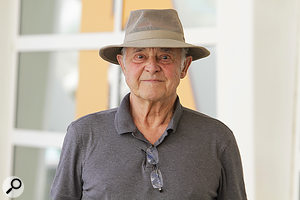John Chowning (at the 2015 Vox Festival). Photo courtesy Histeria.How many people can claim to have discovered an entirely new form of sound synthesis? John Chowning can. He did so by accident while experimenting with different types of vibrato at Stanford University in the mid-'60s. Chowning found that when the frequency of the modulating signal increased beyond a certain point, the vibrato effect disappeared from the modulated tone, and a complex new tone replaced the original. With hindsight, we can see that he had stumbled upon what is now the most common encoding technique used for public radio transmission (hence 'FM' radio). But what made his discovery so serendipitous was that unlike radio engineers, who work at very high frequencies, way above the limits of human hearing, Chowning was able to listen to the modulated waveform. He quickly discovered that FM is a very powerful method of synthesis and, in 1966, became the first person to compose and record a piece of music using FM as the exclusive means of sound generation.

Chowning and his associates spent the next few years refining FM, and laid down a sound mathematical and practical basis for the results they were achieving. Chowning then had the Stanford University Licensing Office approach a number of American manufacturers to see whether they would be interested in implementing it as a commercial method of synthesis. At a time when the Minimoog and ARP Odyssey ruled, polysynths were but a twinkle in electronic engineers' eyes, and 4-bit microprocessors were state-of-the-art devices, none of the American manufacturers saw the potential of FM. So it was almost in desperation that Stanford turned to Yamaha. An engineer named Mr. Ichimura was duly despatched to see Chowning, and the rest, as they say, is history.

As a direct consequence of Yamaha's amazing success throughout the '80s — the company sold millions of FM synthesizers, organs and home keyboards — we now think of FM as an exclusively digital process. But that is not the case. It is more practical to implement it in digital form, but the theory of FM is just as applicable to analogue oscillators, as we shall see...

## Once More, A Little Maths

Last month I explained Amplitude Modulation, and described some of the ways in which it allows you to create new sounds. Let's recap a little. Equations 1 and 2 show two instances of the simplest waveform: a sine wave. The instantaneous amplitudes of the waveforms (their levels at any given point in time, called 'A') are related to their gains (the maximum amplitude reached in their cycles, called 'a'), their frequencies ('w') and time ('t'). And, as before, the subscripts '1' and '2' denote waveform 1 and 2 respectively.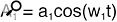Equation 1: A simple 'cosine' wave.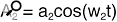Equation 2: A second 'cosine' wave.

If you refer back, you'll also remember that we defined the maximum amplitude of the first waveform as the gain of a VCA, and we modulated this using the second waveform, as shown in Figure 1, below. Equation 3 shows how I wrote the mathematics that describes this arrangement.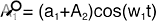Equation 3: The equation defining the output waveform from the VCA in Figure 1.

But now we're going to mix things up a bit. Instead of modulating the amplitude of the first waveform, we're going to modulate its frequency. The block diagram that describes this is deceptively simple, as I have shown in Figure 2. Similarly, the equation describing Frequency Modulation (Equation 4) looks no more fearsome than that describing Amplitude Modulation. Indeed, if you look closely you can see that exactly the same terms are present in both, it's just that one of them (A2) has changed position.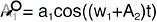Equation 4: The equation defining the output waveform from Oscillator 1 in Figure 2.

If we now echo last month's article and substitute the full expression for A2 into Equation 4, we obtain Equation 5.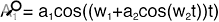Equation 5: Another way of writing Equation 4.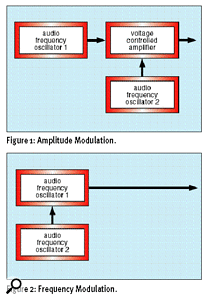Figure 1: [top] Amplitude Modulation. Figure 2: [bottom] Frequency Modulation.At this point, you would be completely justified in running, screaming, for the hills. Unlike last month's equations (which we could interpret using no more than 'O'-level or maybe 'A'-level maths) this one is a monster. Indeed, even with a degree in mathematics, you would be hard pressed to do much with it. You will, therefore, be delighted to know that I'm not even going to try to solve the equation. Unfortunately, that means that you will have to take many of the following facts on trust. But hey... trust me, I once lived with a doctor!

## FM Is Simply Very Fast Vibrato...

Now let's jump back two months, and return to Part 10 of Synth Secrets and the simple vibrato that I described in that article. Figure 3 shows what happens to a waveform (a 'Carrier') whose frequency is being swept up and down by a source of modulation (a 'Modulator'). In this example, the frequency of that Modulator is significantly lower than that of the Carrier.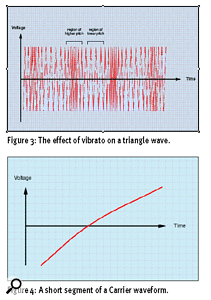Figure 3: [top] The effect of vibrato on a triangle wave. Figure 4: [bottom] A short segment of a Carrier waveform.Now let's ask ourselves what happens as we increase the Modulator's frequency until it approaches, equals, or even exceeds that of the Carrier. At some point, instead of looking like a cyclical 'squeezing' and 'stretching' of the Carrier waveform, the modulation will become a form of distortion within the individual cycles of the Carrier waveform. To demonstrate this I have drawn an example using a very short segment of Carrier waveform — maybe one-eighth of a cycle or thereabouts (Figure 4).

Let's now apply a Modulator to this. In this example it will have a low amplitude, but will be many times the frequency of the Carrier (see Figure 5) — since there are more than seven cycles of modulation in Figure 5, which shows one-eighth of a Carrier cycle, this means that the Modulator frequency is approximately 60 times that of the Carrier. As you will appreciate, this sounds nothing like vibrato. But what does it sound like?

## Side Bands, Side Bands Everywhere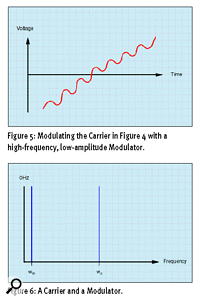Figure 5: [top] Modulating the Carrier in Figure 4 with a high-frequency, low-amplitude Modulator. Figure 6: [bottom] A Carrier and a Modulator.If you refer back to Equation 5, you'll see that the equation for A1 has two 'alien' terms within it: a2 and w2. These are, of course, the gain (maximum amplitude) and the frequency of the Modulator. So it's fair to assume that each of these will have an affect on the nature of the modulated signal. Let's look first at w2, and see what attribute of the output is influenced by the Modulator's frequency.

John Chowning discovered that FM, like AM, generates side bands — additional components, not necessarily harmonically related to the frequency of the Carrier or Modulator — in the frequency spectrum of the output signal. (For an explanation of what side bands are, please refer back to last month.) To see how frequency modulation produces side bands, let's take an example of a sine wave Carrier with frequency wc and a sine wave Modulator of frequency wm. I have shown these in Figure 6 (right).

So far, so good... However, whereas AM generates just two side bands (wc+ wm) and (wc- wm), FM produces a whole series that we can express as follows: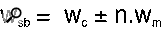Equation 6: The side-band frequencies, where wsb = the series of side-band frequencies, wc = Carrier frequency, wm = Modulator frequency, and n = any integer (0, 1, 2, 3, 4, and so on).

To put this in English: each side band lies at a frequency equal to the Carrier frequency plus or minus an integer multiple of the Modulator frequency. Of course, since 'n' can take any integer value, in theory, applying frequency modulation to a signal produces an infinite series of side bands. In the real world, however, no system has infinite bandwidth, and analogue systems are limited to producing side bands within their finite bandwidth (see later for more on bandwidth). Similarly, manufacturers of digital FM systems constrain the mathematics to those values that they deem significant.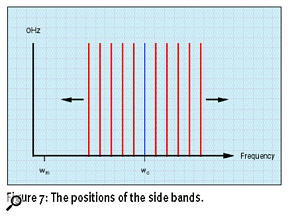Figure 7: The positions of the side bands.Fortunately, and despite this possible complication, the simple formula in Equation 6 makes it easy to see where the side bands are located. Given the Carrier and the Modulator shown in Figure 6 (above), we can show the side bands as shown in Figure 7.

Now, what about the amplitudes of these side bands? OK, we now know that frequency modulation generates side bands, and that the Modulator's frequency determines where they lie. But what is the 'shape' of the resulting spectrum? To answer this, we must turn to the second attribute of the Modulator — its gain, a2 — and introduce a new concept called the 'Modulation Index', or simply 'ß' ('beta').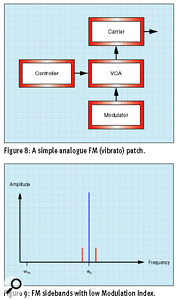Figure 8: [top] A simple analogue FM (vibrato) patch. Figure 9: [bottom] FM side bands with low Modulation Index.To explain ß, I must again direct you to our example of simple vibrato. Imagine a simple synthesizer patch in which the amplitude of a modulation is modified by a VCA that is itself controlled by a Control Voltage source (see Figure 8). Don't forget that in this case, the Modulator frequency wm is very much lower than the Carrier frequency wc.

Consider the case where the gain of the VCA is zero. Clearly, there will be no modulation, and the Carrier will produce a simple, unmodified tone. Now let's increase the gain of the VCA slightly. As you would expect, a gentle vibrato results, much like that of the aforementioned guitarist or violinist. But let's not stop here, and keep increasing the gain until the Modulator is sweeping the Carrier over a wide range of frequencies. At this point, a banshee wail or siren-type sound results. What we learn from this is that the sound we hear is not only determined by the frequency of the Modulator, but also by its gain or maximum amplitude.

Applying these ideas to audio-frequency FM, we must first define ß as the ratio of the Carrier's frequency sweep (the amount by which the Carrier deviates from its unmodulated frequency) divided by the Modulator frequency. We write this as follows: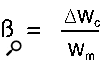Equation 7: The Modulation Index, where ß = the Modulation Index, (pronounced 'delta') means "the change in...", wc = Carrier frequency, and wm = Modulator frequency.

Since the numerator of this expression (the bit 'above the line') is the change in the Carrier frequency, this means that ß is directly related to the amplitude of the Modulator. Now, this is the point at which things get a little weird, because for any given Modulator frequency, it is the Modulation Index (and, therefore, the amplitude of the Modulator) that determines the amplitude of each of the components in the spectrum of the output signal. No, I can't demonstrate why this is so without invoking some of that scary maths I mentioned before, but I can show you a couple of examples.

Let's take that case where the Modulation Index is low — say in the region of 0.1 or less. The only significant side bands will be those closest to the Carrier frequency, and the result will look similar to that we obtained last month using Amplitude Modulation (see Figure 9, above). In contrast, if ß is significantly higher — say, in the region of 5 — we obtain a much broader series of side bands, and a much more complex spectrum results (see Figure 10).

I have shown the first six side bands created by ß=5, but there are far more in the real signal. What's more, you should note an interesting consequence of this value of ß: the amplitude of the original Carrier frequency has diminished significantly. Indeed, there is a value of ß that will cause it to disappear altogether!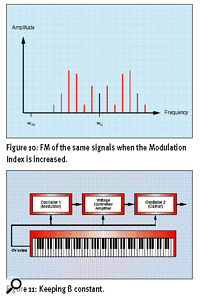Figure 10: [top] FM of the same signals when the Modulation Index is increased. Figure 11: [bottom] Keeping ß constant.Now look at Equation 7 again and you'll see that the denominator (the bit 'below the line') is the frequency of the Modulator. The consequences of this are very far-reaching. Let's say that you have decided that the spectrum in Figure 10 is the tone that you want, and that you want to be able to play the sound up and down the keyboard in conventional manner. This will require both the Carrier and the Modulator to track the keyboard equally so that the harmonic relationship between the spectral components (the side bands) remains constant. But Equation 7 demonstrates that, as the Modulator frequency increases, ß decreases. For example, if you play one octave higher, wm doubles and ß is therefore halved. To avoid this change in the spectrum, the Modulator amplitude must increase proportionally — it must double — to keep ß constant. This is not as much of a problem as it sounds, and Figure 11 shows the simplest way to achieve this.

Mind you, this configuration is almost impossibly difficult to calibrate perfectly, and the vagaries of analogue components ensure that it will, at best, be inconsistent. This is the reason why FM is almost always implemented using digital technology.

## FM Versus Analogue Filters

To move on, we need to talk about bandwidth for a moment. I first mentioned this concept in regard to Equation 6, when I said that real-world systems could not handle a signal of infinite bandwidth. So let's discuss what the real bandwidth of an FM'd signal might be.

For the purposes of this discussion, you could define the bandwidth as the range of frequencies occupied by any given signal. So, for example, a precise sine wave of, say, 100Hz would have negligible bandwidth (it exists only at a specific frequency) whereas a waveform with the same fundamental frequency plus one harmonic at 200Hz would occupy 100Hz of bandwidth. Likewise, a signal occupying the range between 100Hz and 1500Hz would have a bandwidth of 1400Hz, and so on. Now let's apply this concept to the output from an FM system.

Suppose that the Carrier is a sine wave of frequency 500Hz and the Modulator is a sine wave of frequency 300Hz. Clearly, if you mixed these together using a simple audio mixer, then using the simple definition above, the resulting signal would occupy a bandwidth of 200Hz.

Now let's configure the signals so that Amplitude Modulation occurs. From last month we know that the resulting three components have frequencies of wc, wc+ wm, and wc- wm. These frequencies are 500Hz, 200Hz, and 800Hz respectively, so the bandwidth of the resulting signal — the 'spread' between the lowest and highest side bands — is 600Hz.

Now let's consider the bandwidth of an FM'd signal. Although theoretically infinite (remember, the series of side bands is infinite) the Modulation Index will ensure that side bands of higher 'n' are of negligible amplitude. This means that the bandwidth is, to all intents and purposes, finite. Moreover, there is a 'rule-of-thumb' equation that gives us a rough idea of the meaningful bandwidth of the output signal. I have shown this in Equation 8.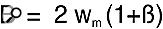Equation 8: The bandwidth of the modulated signal, where B = bandwidth, wm = modulator frequency, and ß = Modulation Index.

Let's say that ß is very small. Then, in our example of a 500Hz Carrier and a 300Hz Modulator, the bandwidth of the output will be equal to 2 x 300Hz x (1 + 0) = 600Hz. Thus, as I stated earlier, for low values of ß the result is much like that obtained using Amplitude Modulation.

But now let's suppose that ß=5. Then, in our example, the bandwidth of the output will be 2 x 300Hz x (1 + 5) = 3,600Hz. Clearly, high values of ß allow FM to create much more complex signals with a much higher bandwidth than the other methods of making two signals interact (see Figure 12, below). Note that this calculation also tells you that, in this example, there are 24 discrete spectral components in the output. Unfortunately, you'll have to read next month's Synth Secrets to find out why.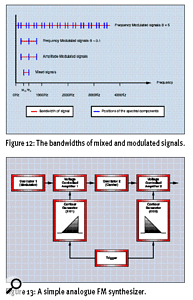Figure 12: [top] The bandwidths of mixed and modulated signals. Figure 13: [bottom] A simple analogue FM synthesizer.Now let's remind ourselves that, in simple subtractive synthesis, changes in the volume of a sound are most often determined by a contour generator acting upon an amplifier. Similarly, any changes in the tone of a sound are usually determined by a contour generator acting upon the cutoff frequency of a filter.

In an FM configuration, the volume of the sound is still determined by the volume envelope of the audio signal (which is, of course, the modulated Carrier), but you no longer need a filter to modify the tone. This is because, for any given Modulator frequency, the Modulator amplitude determines the bandwidth of the output. You can create an interesting demonstration of this using just seven synthesizer modules patched together as shown in Figure 13.

As you can see, the amplitude of the output signal will decrease as EG2 decreases the gain of VCA2 — so over time, the output gets quieter and quieter. At the same time, the maximum amplitude of the Modulator signal derived from VCO1 will increase as EG1 increases the gain of VCA1. This means that, as time passes, the Modulation Index increases, the bandwidth increases, and the output gets brighter and brighter. This is in marked contrast to natural sounds, where increased loudness almost always goes hand-in-hand with increased brightness.

You might think that there's nothing stopping you duplicating this effect using a filter, and it's true that you can use a contour generator and a low-pass VCF to brighten a signal as time passes. But that configuration would be quite incapable of recreating the complex tonal changes that also occur in the FM'd tone — changes that you cannot reproduce using conventional subtractive methods.

## Summary

So let's summarise. Without solving the mathematics of FM, we can say the following two things about the relationship between the Modulator and the output signal:

• The number of significant spectral components and their amplitudes are determined by the Modulation Index, which is proportional to the Modulator's amplitude; but inversely proportional to the Modulator's frequency...

...and...

• For any given Carrier frequency, the position of the spectral components is determined by the Modulator's frequency alone.

Although the proof of these statements and the calculation of the side bands' amplitude spectrum is a nightmare, the basics are easily understood. And, whether we perform FM using an analogue synth or a digital one, these principles remain the same. So here's this month's Synth Secret (which, as usual, is no secret at all):

Frequency Modulation is a powerful method of synthesis that is as relevant to analogue synthesizers as it is to digital ones, and which is capable of generating sounds unobtainable by any other method.

Next month, we'll take a closer look at some practical aspects of Frequency Modulation, and introduce the idea of 'operators' — a common concept if you own a DX7 synthesizer, but perhaps not one that you have encountered in analogue technology. We'll even take a look at some basic FM programming on a modular analogue synth.

Until then...